# ข้อสอบ BMAT Part2 พร้อมเฉลย มาทดสอบกันพร้อมสอบแค่ไหน?

## BMAT Part2

1. DNA is the class of biomolecules that contains genetic information of living organisms. Which statements are true about the DNA of a cell?

1)Nuclear DNA contains the entire genetic information of an animal cell.
2) DNA is replicated during the interphase of the cell cycle before mitosis.
3) The ratio between A and T in a strand of DNA is 1:1.
4) Mutation of DNA base sequence during meiosis is a source of phenotypic variation.
2. Following the statement

i) Fe(ClO2)3 and K2[Fe(OH)4] have the same oxidation number which is +2.
ii) The solution to find the oxidation number of S and O atoms in SO2 is the first step should be finding S atom which is -2 because S has the highest EN in this compound.
iii) NaOH + Cl2 → NaCl + NaClO3 + H2O. In this reaction, Chlorine has oxidation state -1 in NaClO3
iv) Disproportionation reaction is an element in one oxidation state that is simultaneously oxidized and reduced.
v) The reaction 2NO2 + H2O → HNO2 + HNO3 is a disproportionation reaction.

How many choice(s) is/are correct?
3. An object of mass 280 g thrown at a certain angle from Earth's surface to reach a maximum height at 80 cm and falls back to Earth. Assume there is no air resistance, and the velocity becomes zero when it hits the ground. If the initial horizontal speed of the object is 3.0 m/s, which of the following statements must be CORRECT?

P) The initial speed of the object is 5.0 m/s
Q) The horizontal distance when the object returned to the initial level is 2.4 m
R) The change in momentum when the object hits the ground is 2.0 N·s
4. Which of the following is a simplification of
((x - 1)/(x - 2)) - ((x2 - 2x + 3)/(x2 - x -2)) ?
5. Some mice have a recessive genetic condition that has a 50% infant mortality rate.

Those that survive lose the ability to reproduce.

Two heterozygous mice mated to produce some offspring. One of the offspring of unknown genotype mated with another heterozygous mouse and produced a total 24 offspring.

Assuming there were no other mortal conditions, how many of the offspring were expected to have died in their infancy?
6. The figure shows a circuit that contains three resistors. The resistors R1 and R2 are identical, and the powers dissipated from R1 and R3 are P1 and P3, respectively. If the R2 is now doubled while the others stay the same, what would be the now powers dissipated from R1 and R3?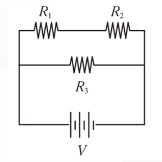7. Some elements of the electron configuration in the ground state

 A element B element C element 2  8 2  8  2 2  8  7

Consider the information of A, B, C elements below,
1. Element A is low reactive because of high stability.
2. The ionization of element A is higher than element C.
3. The oxide compound of element B has the empirical formula that is B2
4. Element B is group 2A and period 3, and element C is group 5A and period 3.
5. Element A is the gas state which is used for the fluorescence lamp.

Which statement is incorrect?

8. A, B, and C are points on a circle that has its centre point at O.

A is at (-6, 5), B is on the y-axis, and C is at point (0, -1).

AC is the diameter of the circle.

Line CQ is a segment of the line 5y = 3x - 5, and point O is on line BQ.

What is the value of 2b - a if the coordinates of Q are (a, b)?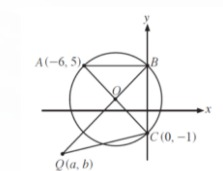9. An athlete is training for a marathon event. His heart rate and stroke volume increase significantly higher than when he is at rest.

Which one of these statements explains these changes?

10. Vulcanization is the process of crosslinked rubber which makes rubber has an elastic property. The structure of rubber is a tangle of polymeric chains, and if the external force is strong enough, individual chains slip past one another. Therefore causing the rubber to lose most of its elasticity.

This process was discovered by Charles Goodyear in 1839 that Sulfur (S8) and ZnO as catalyst can be linked at double bonds between chains of natural rubber polymer.

Following the figure.

What is a type of bond of crosslinked rubber?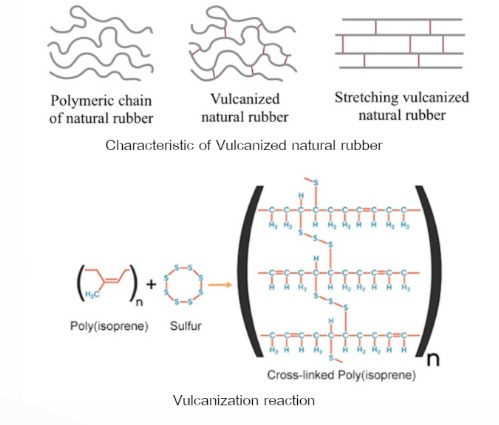11. An elevator is supported by a cable that connects to an electric motor. The elevator of mass of 783 kg is lifted vertically through a height of 22.0 m in a time of 180 s. If the motor is connected to a constant 110 V D.C. supply and the complete lifting system is 87% efficient.

What is the current in the electric motor? (Given gravitational field strength = 10 m⋅s–2)

12. ABCDEF is a regular hexagon of side. Find the shaded area in cm2 ?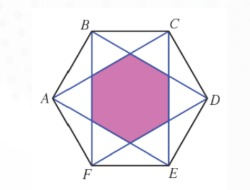13. Substances can be transported through different processes in both biological and non-biological systems.

Which row describes the processes correctly?

14. 8.28 grams of Lead metal sheet reacts with 1.2 M, 50 cm3 of Silver nitrate, in the Erlenmeyer flask is found Silver precipitate which has 3.24 grams and the reaction causes in the below.

Pb (s) + AgNO3 (aq) → Ag (s) + Pb(NO3)2 (aq)

What is the method to find the limiting reagent and how many percent yields of Silver precipitate? (Ar values: Pb = 207 ; Ag = 108 ; S = 32 ; O = 16)

15. An orange light ray with wavelength of 620 nm passes from air into liquid. Its speed decreases by 25%. What is the wavelength and the color of the light beam in the liquid?

16. To go to his school, Tony first catches a bus and then catches a boat.

The probability that the bus will be on time is 0.6.

The probability that the bus will be late is 0.4.

If the bus is on time, then the probability that he will catch the boat is 0.8.

If the bus is late, then the probability that he will catch the boat is 0.6.

Given that Tony catches the boat, what is the probability that the bus was on time?

17.The contents of the blood vary according to the current health and conditions of the body. Which one of these statements is NOT true about blood contents?

18. The reaction of A + B → C has 2 steps. Following the graph between potential energy and the reaction process.

and following the statement
1. If the catalyst is added into the reaction, the activation energy of this reaction will be lower than c.
2. The energy of reaction should be A + B → C + c
3. S is an intermediate compound and T is a catalyst.
4. The stability of X and Y is higher than C.
5. Increasing the temperature will decrease the activation energy of this reaction.

Which of the statements is true?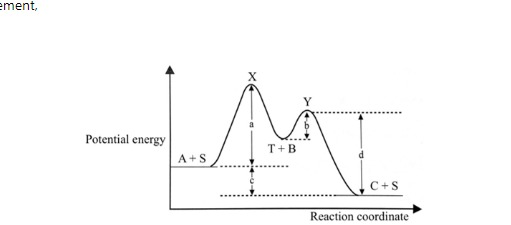19.Which statement(s) below is(are) INCORRECT?

P) In a wood-fired pizza oven, the heat is transferred to the pizza only by convection.

Q) Using a curling iron to curl hair, the heat is transferred to the hair only by conduction.

R) If croissants are reheated in a microwave, most heat is transferred to the bread by radiation.

S) An object is floating in a vacuum chamber. When the rate of radiation emission is equal to the rate of radiation absorption; the object is at the same temperature as its surroundings.

20. A sample initially contains 8N and 2N atoms of radioactive isotopes X and Y, respectively. Both radioactive isotopes X and Y decay in a single stage into a stable isotope Z. If the isotopes X and Y have half-life of 15 minutes and 30 minutes, what is the ratio number of atoms of X: number of atoms of Y: number of atoms of Z in the sample an hour later?
21. Embryonic stem cells have great potential in research and therapy as they have the ability to differentiate into almost all cell types in the body. Embryonic stem cells are obtained from early embryos of mammals.

Which one of the statements is NOT true regarding the generation of a mammalian embryo and the uses of embryonic stem cells?

22. Concentrated aqueous copper (II) bromide was electrolyzed. After a few minutes, the remaining electrolyte solution was tested with a pH probe at 25 °C.

The gases produced at the electrodes were collected and tested with a colorless aqueous solution of sodium iodide.

Which row in the following table best describes the observations in these tests?

 the pH of the remaining solution test of gas from anode (positive electrode) test of gas from cathode (negative electrode) A. 3 no observable change violet solid B. 3 violet solid no observable change C. 7 no observable change violet solid D. 7 violet solid no observable change E. 10 no observable change violet solid F. 10 violet solid no observable change
23. Ecosystems are the combination of living organisms and their non-living environment. Both living organisms and their environment are dynamic and ever-changing due to both natural and human-made causes.

Which statements are true regarding how living organisms and their environment changes over time?

1. Deforestation decreases the amount of carbon dioxide converted by producers into biomass.
2. An individual of a species evolves over time by natural selection as the environment changes.
3. Recombinant DNA technology can contaminate natural population with undesirable genes leading to imbalances in food webs.
4. The emergence of a disease can decrease the size of a population while increasing the size of another population living in the same habitat.

24. Beta-carotene is an orange-red natural dye that is extracted from many orange-red fruits and vegetables and Curcumin is a yellow natural dye extracted from Turmeric. Both compounds are natural dyes that do not react with each other and do not react with Methanol and Hexane.

Following the structure.

If the two natural dyes are mixed in the beaker which has the mixed solvent between Hexane and Methanol, what observation in the beaker is correct? (given density of C6H14 = 655 kg/dm3 ; density of CH3OH = 792 kg/dm3 and the mass of two natural dyes which is mixed in solvent does not change the density of solvents)

 The observation in the beaker A. The mixture is separated. The orange-red is the top of the beaker and the yellow is below the beaker. B. The mixture is separated. The yellow is the top of the beaker and the orange-red is below the beaker. C. The mixture is separated. The yellow is the top of the beaker and the clear solution is below the beaker. D. The mixture is separated.  The clear solution is the top of the beaker and the yellow is below the beaker. E. The mixture is separated. The orange-red is the top of the beaker and the clear solution is below the beaker. F. The mixture is separated.  The clear solution is the top of the beaker and the orange-red is below the beaker. G. The mixture becomes the orange solution. H. None of above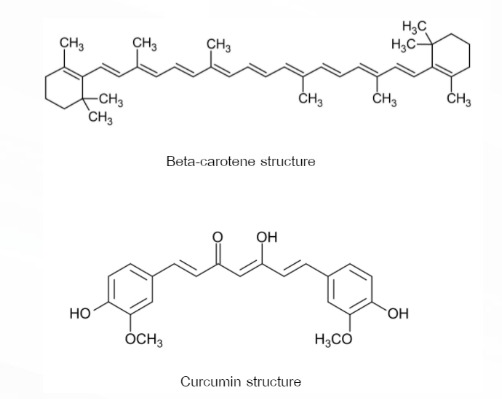25.Consider two uniform spherical planets of equal density but unequal radius.

Which of the following quantities will not change for both planets?

P) The orbital period of a satellite in a circular orbit at a given distance from the planet's center.
Q) The orbital period of a satellite in a circular orbit just above the planet's surface.
R) The acceleration due to gravity at the planet's surface.
S) The escape velocity from the planet's surface.
BMAT Part2
You got {{userScore}} out of {{maxScore}} correct
{{title}}
{{image}}
{{content}}